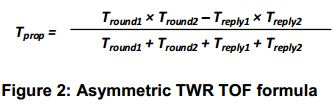# Asymmetric double sided TWR

Hi,

In DW1000 and two-way ranging application note(APS013), how the equation for Asymmetric double sided TWR equation (Asymmetric TWR TOF formula) is derived. Is there any document explains this.Thanks,
Shijo Thomas

1 Like

Hi shijomts,

Please refer Chapter 12 in DW1000 user manual which explains about Asymmetric double sided TWR.

Thanks & Regards,
Deepa

Hi Deepa,

This chapter explains about Asymmetric double sided TWR. But does not show the steps to arrive at the equation.

Here the equation for calculating the Tprop is shown. But does not shows how this equation is derived.

I hate to link directly to a word document but this looks to be the source of the equation (and the diagrams): https://mentor.ieee.org/802.15/dcn/15/15-15-0429-00-0008-text-for-relative-positioning-and-location.docx

The appendix at the end gives the full derivation of the formula.

1 Like

Hi Andy,

Thanks for the support.

Regards,
Shijo Thomas

I was looking for this. Thanks a lot!